Скачать презентацию ICS 312 Set 15 FILE OPERATIONS FILE

64b3e628a711f52dfff2261257dee2b7.ppt

• Количество слайдов: 27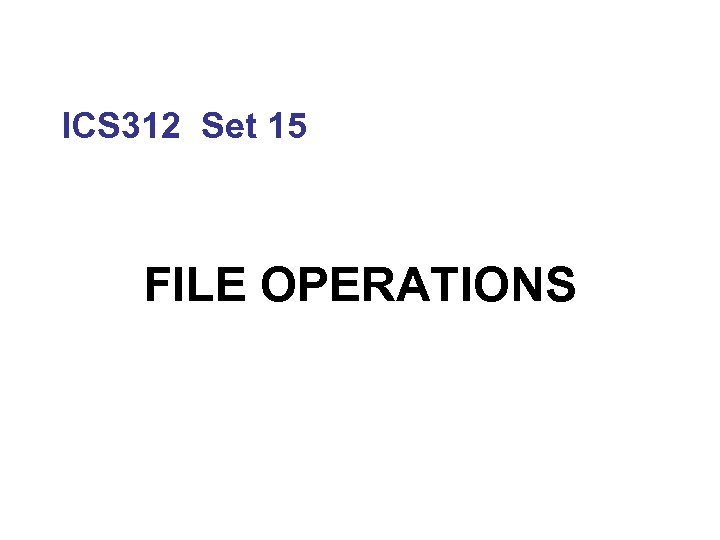ICS 312 Set 15 FILE OPERATIONS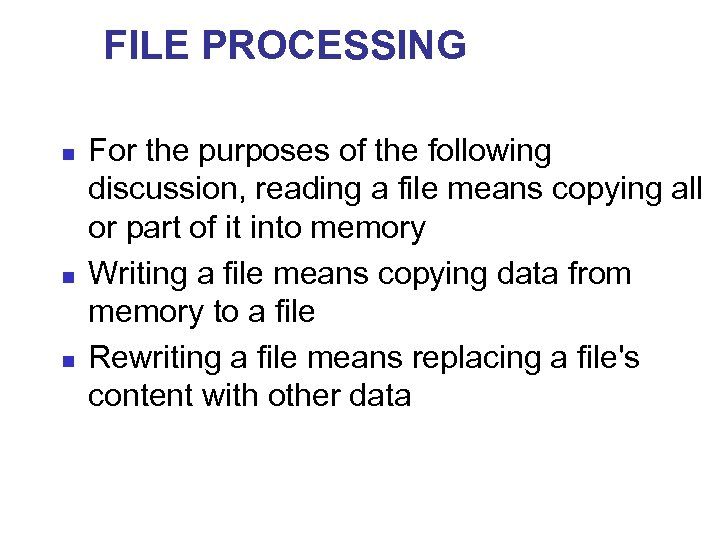FILE PROCESSING n n n For the purposes of the following discussion, reading a file means copying all or part of it into memory Writing a file means copying data from memory to a file Rewriting a file means replacing a file's content with other data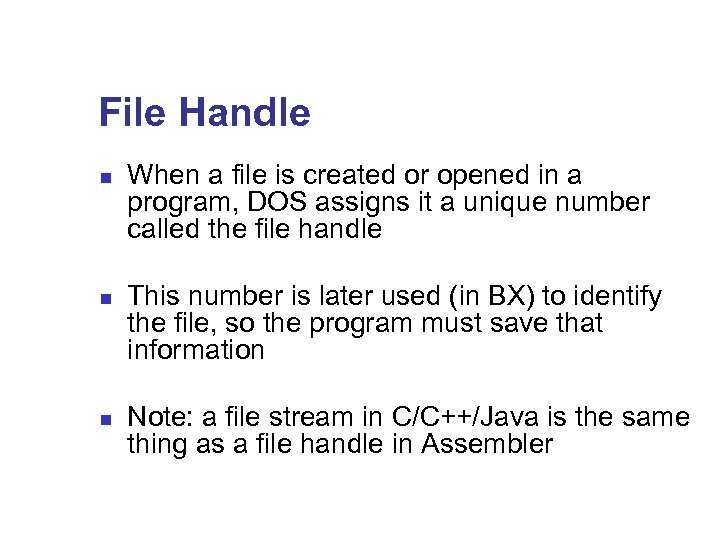File Handle n n n When a file is created or opened in a program, DOS assigns it a unique number called the file handle This number is later used (in BX) to identify the file, so the program must save that information Note: a file stream in C/C++/Java is the same thing as a file handle in Assembler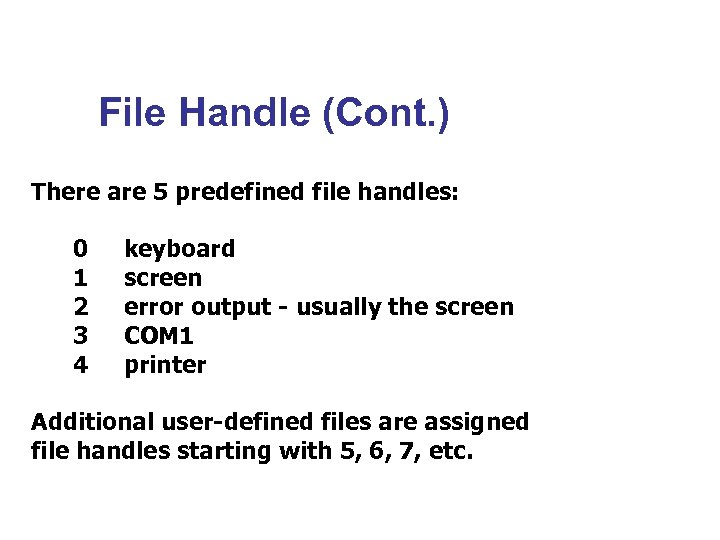File Handle (Cont. ) There are 5 predefined file handles: 0 keyboard 1 screen 2 error output - usually the screen 3 COM 1 4 printer Additional user-defined files are assigned file handles starting with 5, 6, 7, etc.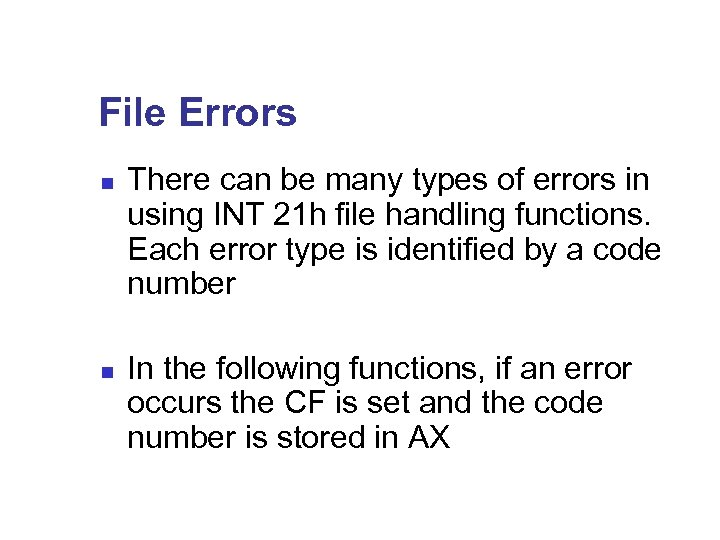File Errors n There can be many types of errors in using INT 21 h file handling functions. Each error type is identified by a code number n In the following functions, if an error occurs the CF is set and the code number is stored in AX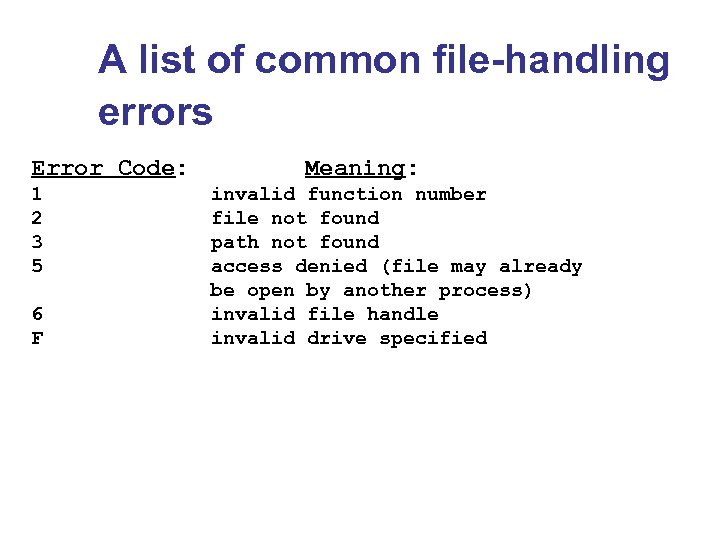A list of common file-handling errors Error Code: Meaning: 1 invalid function number 2 file not found 3 path not found 5 access denied (file may already be open by another process) 6 invalid file handle F invalid drive specified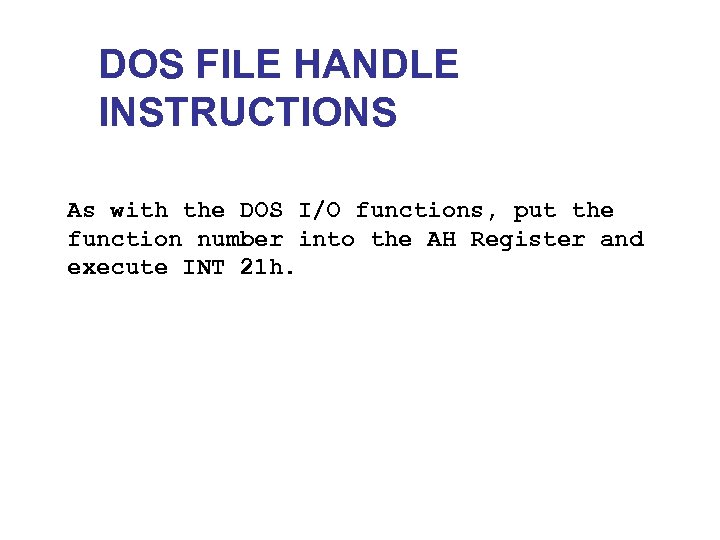DOS FILE HANDLE INSTRUCTIONS As with the DOS I/O functions, put the function number into the AH Register and execute INT 21 h.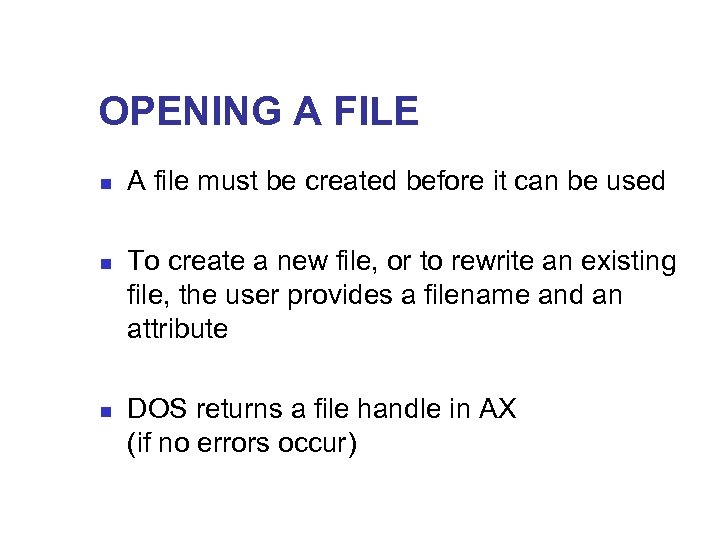OPENING A FILE n n n A file must be created before it can be used To create a new file, or to rewrite an existing file, the user provides a filename and an attribute DOS returns a file handle in AX (if no errors occur)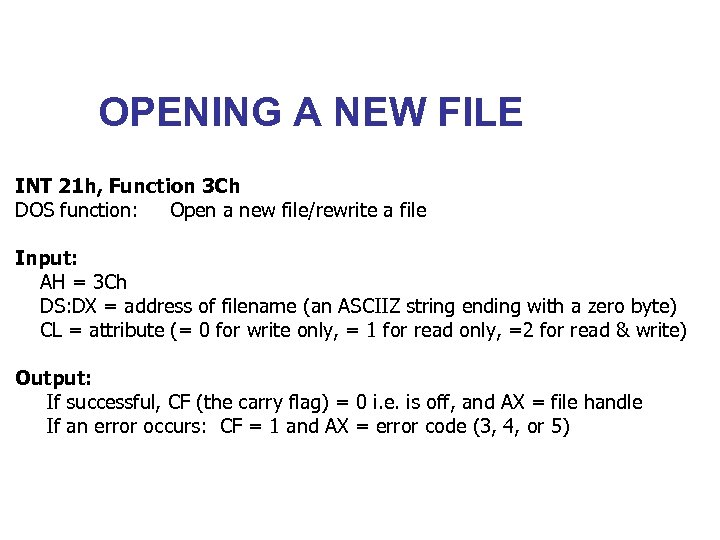OPENING A NEW FILE INT 21 h, Function 3 Ch DOS function: Open a new file/rewrite a file Input: AH = 3 Ch DS: DX = address of filename (an ASCIIZ string ending with a zero byte) CL = attribute (= 0 for write only, = 1 for read only, =2 for read & write) Output: If successful, CF (the carry flag) = 0 i. e. is off, and AX = file handle If an error occurs: CF = 1 and AX = error code (3, 4, or 5)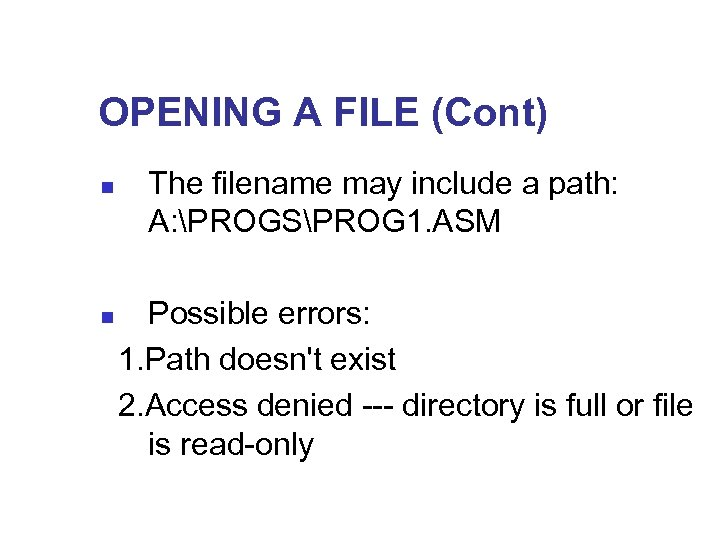OPENING A FILE (Cont) n The filename may include a path: A: PROGSPROG 1. ASM Possible errors: 1. Path doesn't exist 2. Access denied --- directory is full or file is read-only n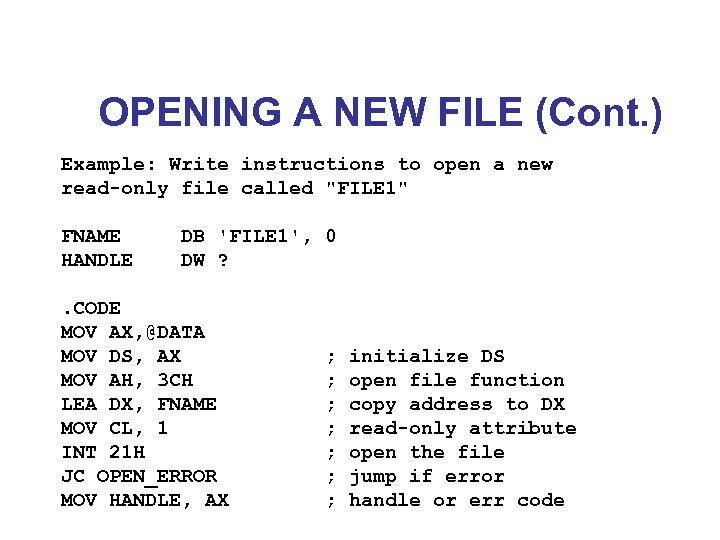OPENING A NEW FILE (Cont. ) Example: Write instructions to open a new read-only file called "FILE 1" FNAME DB 'FILE 1', 0 HANDLE DW ? . CODE MOV AX, @DATA MOV DS, AX ; initialize DS MOV AH, 3 CH ; open file function LEA DX, FNAME ; copy address to DX MOV CL, 1 ; read-only attribute INT 21 H ; open the file JC OPEN_ERROR ; jump if error MOV HANDLE, AX ; handle or err code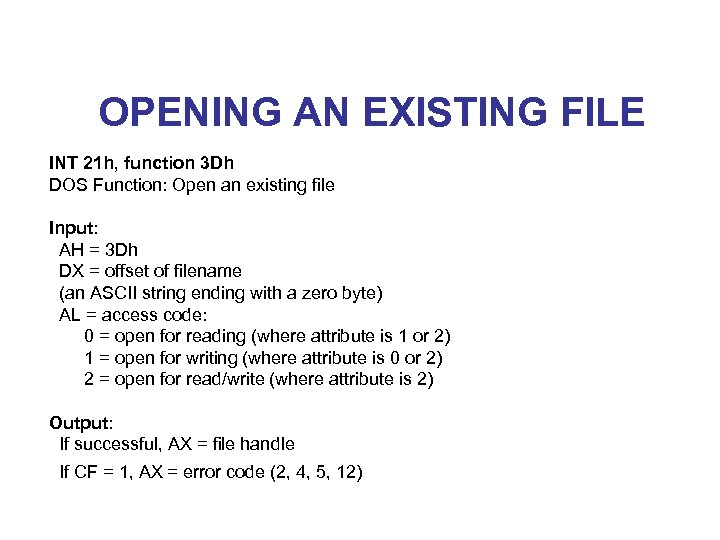OPENING AN EXISTING FILE INT 21 h, function 3 Dh DOS Function: Open an existing file Input: AH = 3 Dh DX = offset of filename (an ASCII string ending with a zero byte) AL = access code: 0 = open for reading (where attribute is 1 or 2) 1 = open for writing (where attribute is 0 or 2) 2 = open for read/write (where attribute is 2) Output: If successful, AX = file handle If CF = 1, AX = error code (2, 4, 5, 12)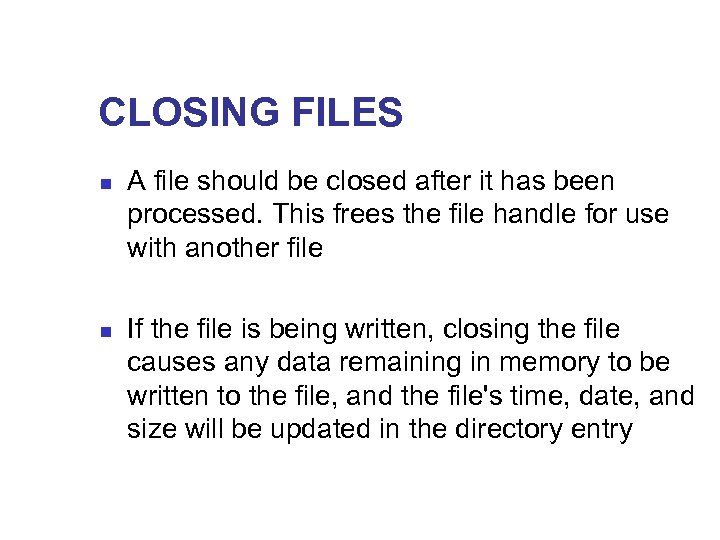CLOSING FILES n n A file should be closed after it has been processed. This frees the file handle for use with another file If the file is being written, closing the file causes any data remaining in memory to be written to the file, and the file's time, date, and size will be updated in the directory entry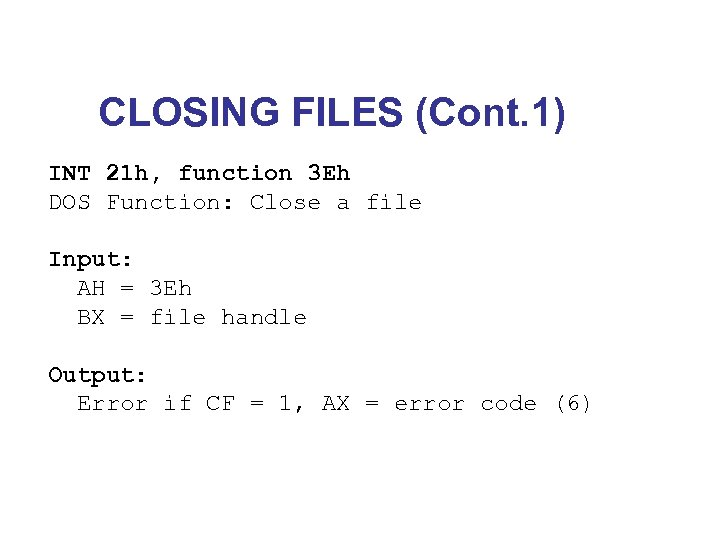CLOSING FILES (Cont. 1) INT 21 h, function 3 Eh DOS Function: Close a file Input: AH = 3 Eh BX = file handle Output: Error if CF = 1, AX = error code (6)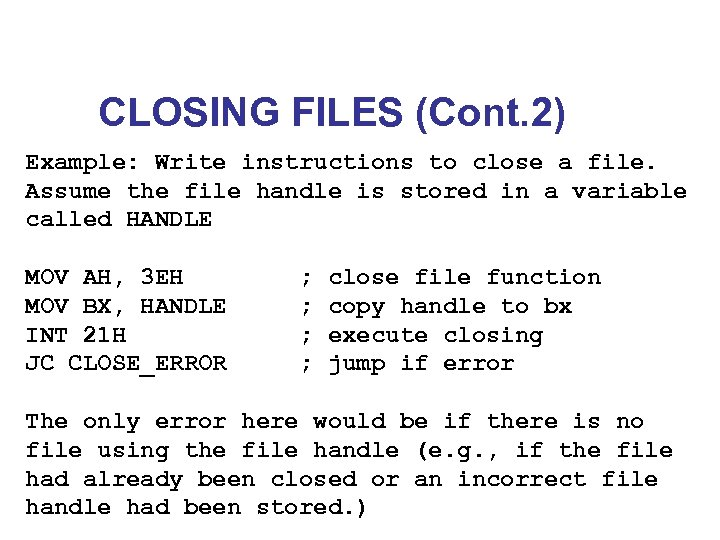CLOSING FILES (Cont. 2) Example: Write instructions to close a file. Assume the file handle is stored in a variable called HANDLE MOV AH, 3 EH ; close file function MOV BX, HANDLE ; copy handle to bx INT 21 H ; execute closing JC CLOSE_ERROR ; jump if error The only error here would be if there is no file using the file handle (e. g. , if the file had already been closed or an incorrect file handle had been stored. )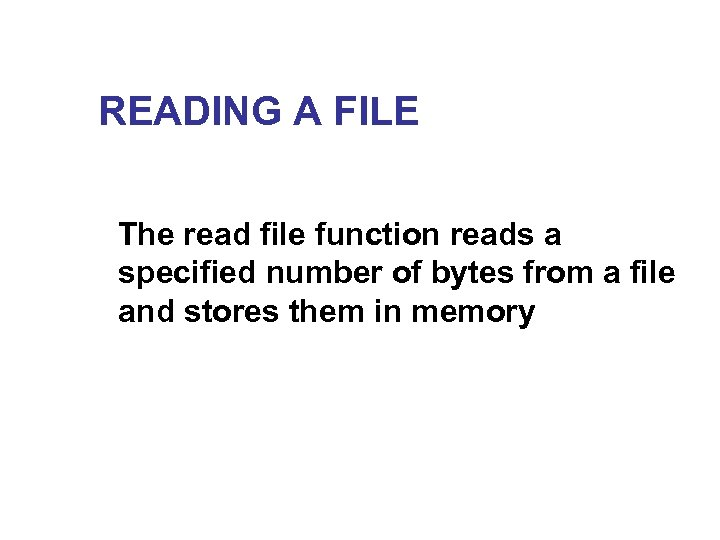READING A FILE The read file function reads a specified number of bytes from a file and stores them in memory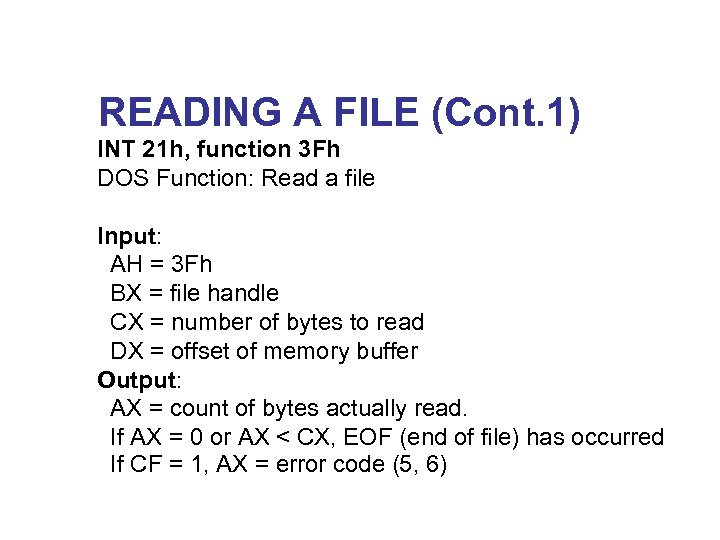READING A FILE (Cont. 1) INT 21 h, function 3 Fh DOS Function: Read a file Input: AH = 3 Fh BX = file handle CX = number of bytes to read DX = offset of memory buffer Output: AX = count of bytes actually read. If AX = 0 or AX < CX, EOF (end of file) has occurred If CF = 1, AX = error code (5, 6)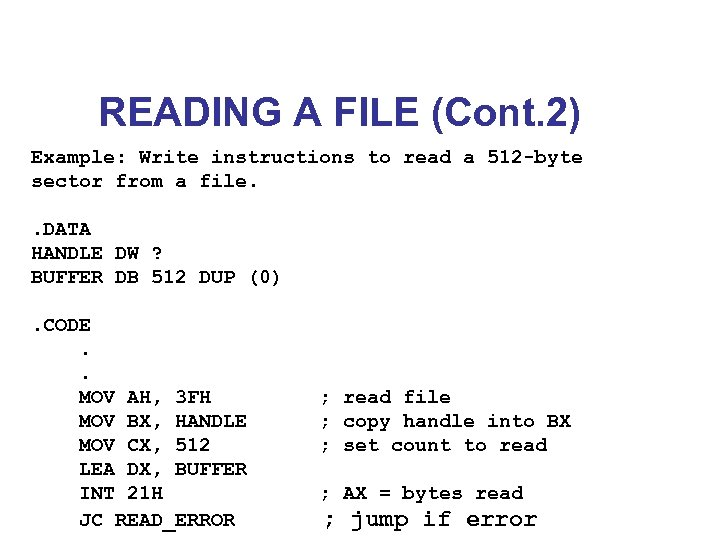READING A FILE (Cont. 2) Example: Write instructions to read a 512 -byte sector from a file. . DATA HANDLE DW ? BUFFER DB 512 DUP (0). CODE . . MOV AH, 3 FH ; read file MOV BX, HANDLE ; copy handle into BX MOV CX, 512 ; set count to read LEA DX, BUFFER INT 21 H ; AX = bytes read JC READ_ERROR ; jump if error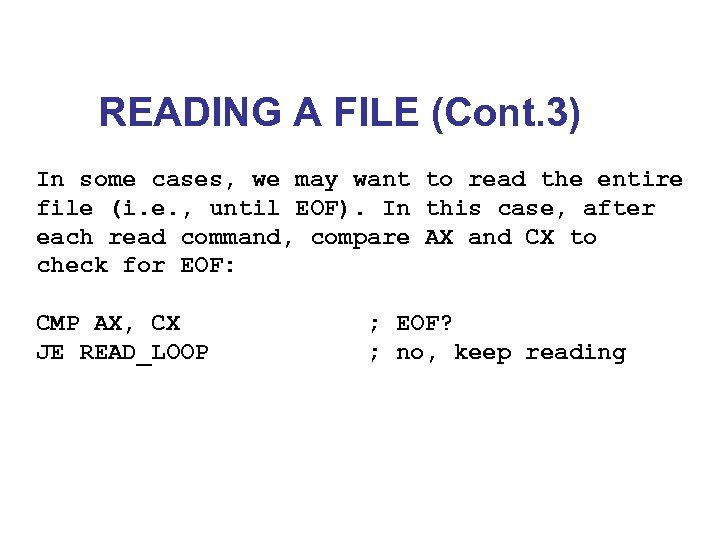READING A FILE (Cont. 3) In some cases, we may want to read the entire file (i. e. , until EOF). In this case, after each read command, compare AX and CX to check for EOF: CMP AX, CX ; EOF? JE READ_LOOP ; no, keep reading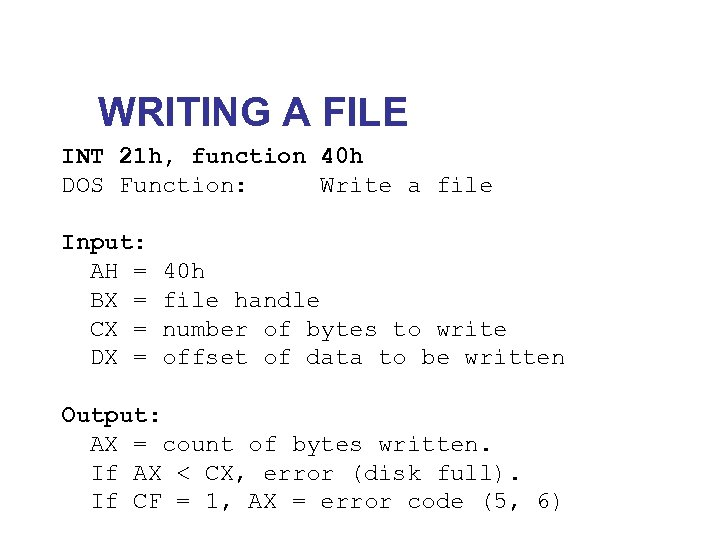WRITING A FILE INT 21 h, function 40 h DOS Function: Write a file Input: AH = 40 h BX = file handle CX = number of bytes to write DX = offset of data to be written Output: AX = count of bytes written. If AX < CX, error (disk full). If CF = 1, AX = error code (5, 6)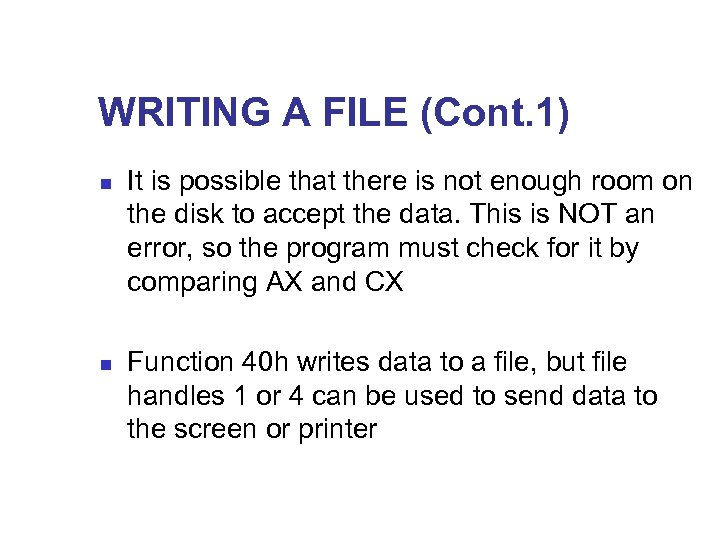WRITING A FILE (Cont. 1) n n It is possible that there is not enough room on the disk to accept the data. This is NOT an error, so the program must check for it by comparing AX and CX Function 40 h writes data to a file, but file handles 1 or 4 can be used to send data to the screen or printer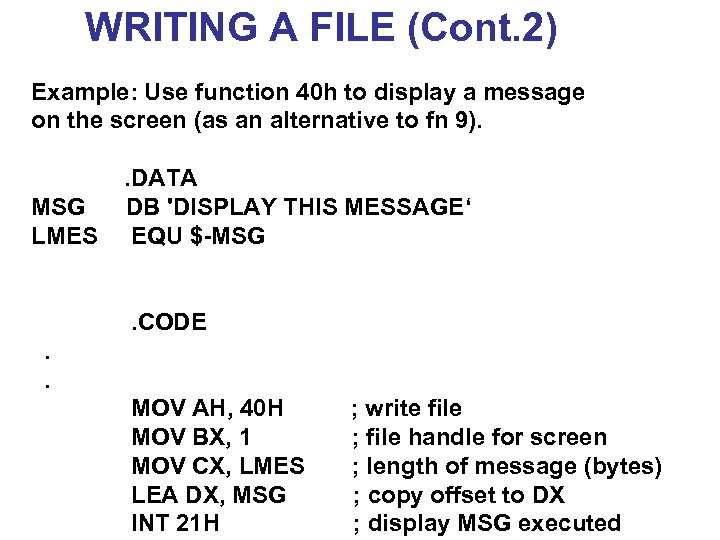WRITING A FILE (Cont. 2) Example: Use function 40 h to display a message on the screen (as an alternative to fn 9). . DATA MSG DB 'DISPLAY THIS MESSAGE‘ LMES EQU \$-MSG . CODE . . MOV AH, 40 H ; write file MOV BX, 1 ; file handle for screen MOV CX, LMES ; length of message (bytes) LEA DX, MSG ; copy offset to DX INT 21 H ; display MSG executed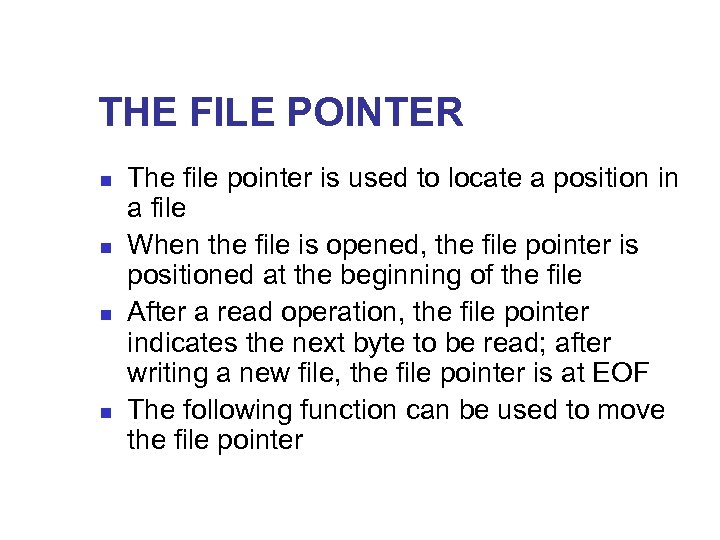THE FILE POINTER n n The file pointer is used to locate a position in a file When the file is opened, the file pointer is positioned at the beginning of the file After a read operation, the file pointer indicates the next byte to be read; after writing a new file, the file pointer is at EOF The following function can be used to move the file pointer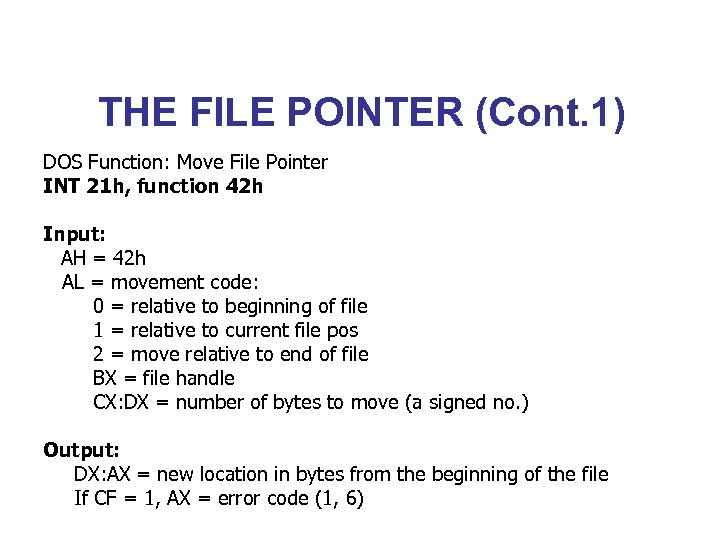THE FILE POINTER (Cont. 1) DOS Function: Move File Pointer INT 21 h, function 42 h Input: AH = 42 h AL = movement code: 0 = relative to beginning of file 1 = relative to current file pos 2 = move relative to end of file BX = file handle CX: DX = number of bytes to move (a signed no. ) Output: DX: AX = new location in bytes from the beginning of the file If CF = 1, AX = error code (1, 6)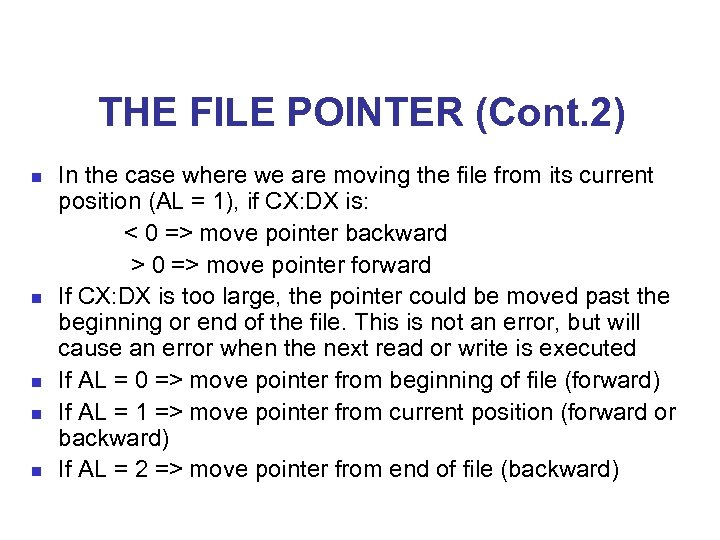THE FILE POINTER (Cont. 2) In the case where we are moving the file from its current position (AL = 1), if CX: DX is: < 0 => move pointer backward > 0 => move pointer forward n If CX: DX is too large, the pointer could be moved past the beginning or end of the file. This is not an error, but will cause an error when the next read or write is executed n If AL = 0 => move pointer from beginning of file (forward) n If AL = 1 => move pointer from current position (forward or backward) n If AL = 2 => move pointer from end of file (backward) n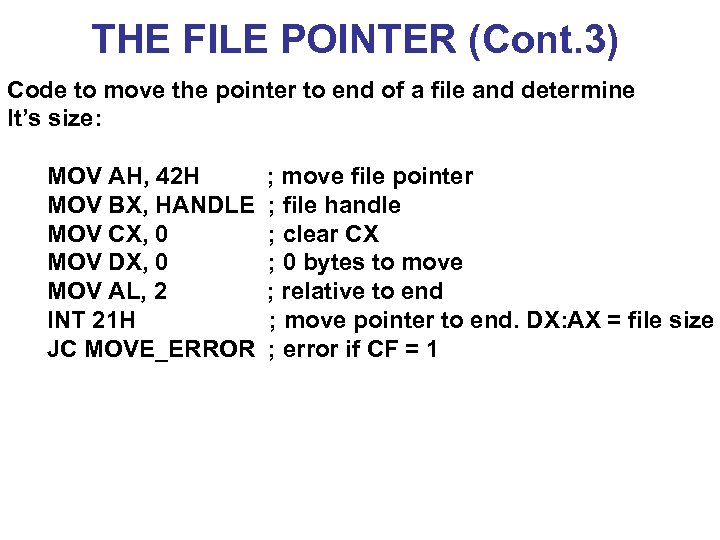THE FILE POINTER (Cont. 3) Code to move the pointer to end of a file and determine It’s size: MOV AH, 42 H ; move file pointer MOV BX, HANDLE ; file handle MOV CX, 0 ; clear CX MOV DX, 0 ; 0 bytes to move MOV AL, 2 ; relative to end INT 21 H ; move pointer to end. DX: AX = file size JC MOVE_ERROR ; error if CF = 1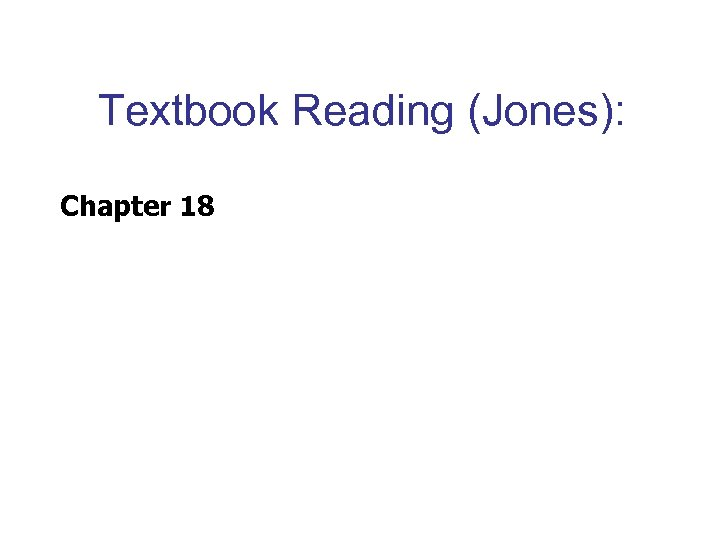Textbook Reading (Jones): Chapter 18